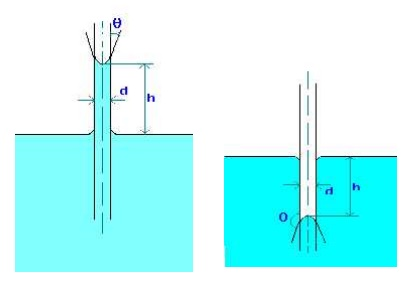Home | | Fluid Mechanics and Machinery | Compressibility, Surface tension, Capillarity

# Compressibility, Surface tension, Capillarity

Compressibility is the reciprocal of the bulk modulus of elasticity, K which is defined as the ratio of compressive stress to volumetric strain.

COMPRESSIBILITY

Compressibility is the reciprocal of the bulk modulus of elasticity, K which is defined as the ratio of compressive stress to volumetric strain.

Compressibility is given by = 1/K

SURFACE TENSION

Surface tension is defined as the tensile force acting on the surface of a liquid in contact with a gas or on the surface between two two immiscible liquids such that the contact surface behaves like a membrane under tension.1.A soap bubble 50 mm in diameter contains a pressure (in excess of atmospheric) of 2 bar. Find the surface tension in the soap film.

Data:

Radius of soap bubble (r) = 25 mm = 0.025 m Dp = 2 Bar = 2 x 105 N/m2

Formula:

Pressure inside a soap bubble and surface tension (s) are related by, Dp = 4s/r

Calculations:

s = Dpr/4 = 2 x 105 x 0.025/4 = 1250 N/m

CAPILLARITY

Capillarity is defined as a phenomenon of rise or fall of a liquid surface in a small tube relative to the adjacent general level of liquid when the tube is held vertically in the liquid. The rise of liquid surface is known as capillary rise while the fall of the liquid surface is known as capillary depression.

It is expressed in terms of cm or mm of liquid. Its value depends upon the specific weight of the liquid, diameter of the tube and surface tension of the liquid.1.Water has a surface tension of 0.4 N/m. In a 3 mm diameter vertical tube if the liquid rises 6 mm above the liquid outside the tube, calculate the contact angle.

Data:

Surface tension (s) = 0.4 N/m

Dia of tube (d) = 3 mm = 0.003 m

Capillary rise (h) = 6 mm = 0.006 m

Formula:

Capillary rise due to surface tension is given by

h = 4   cos(q)/(rgd), where q is the contact angle.

Calculations:

cos(q) = hrgd/(4s) = 0.006 x 1000 x 9.812 x 0.003 / (4 x 0.4) = 0.11

Therfore, contact angle q = 83.7o

Study Material, Lecturing Notes, Assignment, Reference, Wiki description explanation, brief detail
Mechanical : Fluid Mechanics And Machinery : Fluid Properties and Flow Characteristics : Compressibility, Surface tension, Capillarity |

Related Topics